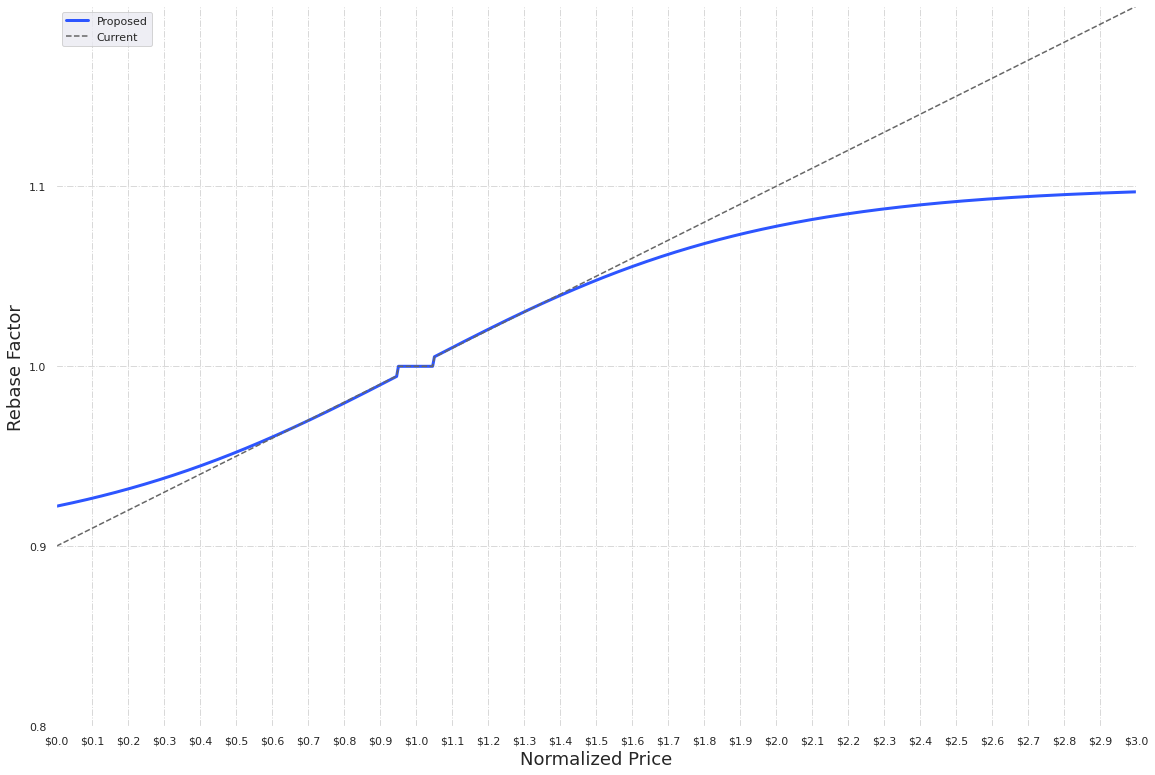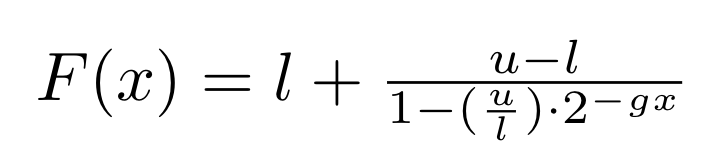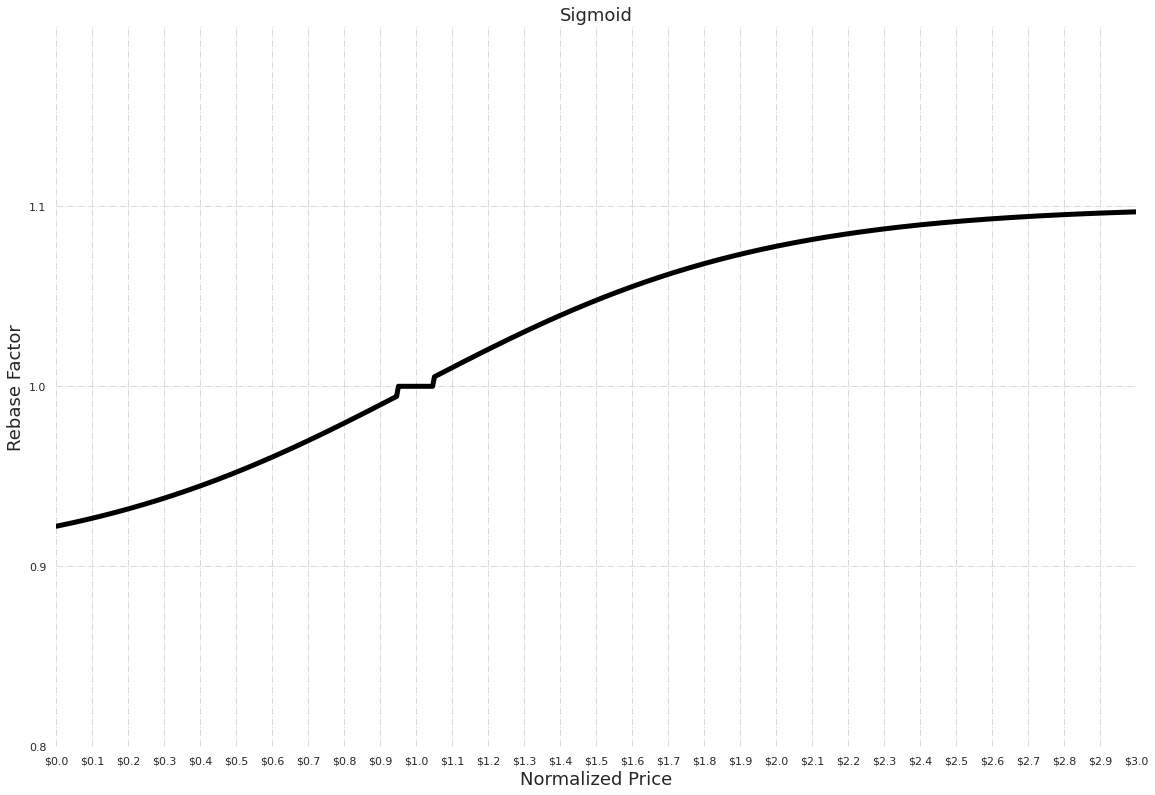# AIP 5: Sigmoid Rebase Function Source

Author Ahmed Naguib Aly https://forum.ampleforth.org/t/rebase-curve-upgrade-proposal/327 Implemented 2020-12-1

## Summary

Change the rebase factor function from a linear curve to an S-shaped curve.

## Abstract

The proposal is to deploy a new contract that updates the current supply policy’s linear rebase function with a Sigmoid function.

## Motivation

The original Ampleforth linear rebase function was intended to make minimal assumptions about how price reacts to expansion and contraction–i.e. expansion would slow price increases by translating them into supply increases and contraction would slow price decreases by translating them into supply decreases. Hence the main purpose of the function is to point supply change in the right direction without trying to predict the exact magnitude of supply change needed for the fastest convergence to the price target.

At this point in time there is history to analyze and use to improve the rebase curve accordingly. e.g, we can see that extreme market scenarios can have outsized effects on AMPL supply, which then require prolonged supply corrections. The linear curve is very limited on how it can be configured and an S-shaped curve can allow for more sophisticated tuning of the rebase function.

The advantages of an Sigmoid curve:

• Can be configured to have different slopes at different points of the curve.

• It has bounds (asymptotes)

• That makes AMPL supply changes relatively more predictable beyond the current day
• Limits the potential effect from a bad oracle price. This makes AMPL more resilient to errors and attacks and also increases the feasibility of further oracle decentralization efforts.

## Specification

The smart contract upgrade replaces the current linear rebasing function with a sigmoid curve that caps supply changes at its asymptotesRebase factor of 1.0 means no rebase.
Normalized price = VWAP price / Target price


The proposed curve above is generated by the sigmoid function:F(x) : fraction of supply added or removed (supply change %)

x : normalized price deviation

NOTE: x in the formula above is normalized price deviation and F(x) is the fraction of change while the diagram for better visual representation shows the normalized price on the x-axis and the supply multiplier factor on the y-axis.

It has shaping parameters that determine: lower asymptote, upper asymptote, and the steepness of the curve (ie: growth rate).

l = lower asymptote
u = upper asymptote
g = growth rate


### Technical Specification

This update creates no changes to external APIs. Clients, including exchanges that listen to AMPL’s rebase events, will still receive the absolute supply change integer as before. However, note that any external application that calculates the supply change independently will need to update their calculation logic.

### Parameter Values

The parameters are chosen to be very similar to the current curve in the normalized price range of ($0.5 to$1.5) and caps the changes to 10% on the expansion side, and that caps the contraction side at -7.78%

The proposed values are:

l (Lower) = -0.1
u (Upper) = 0.1
g (Growth) = 3### Implementation

https://github.com/ampleforth/ampleforth-contracts/pull/226

### Test Cases

Existing unit tests will be updated with the correct calculation results to maintain test coverage.

## Conclusions

The Ampleforth protocol will maintain the same nature to change supply to match demand. And the new curve would allow further improvements by changing the sigmoid parameters, which is independent from this AIP.</p>